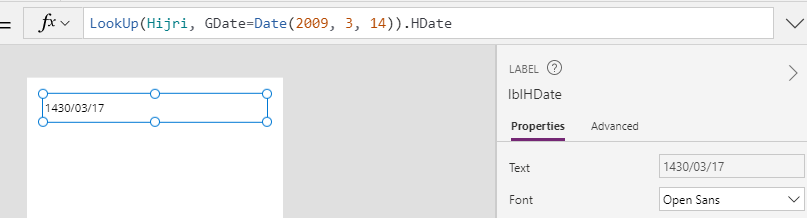# Dates - How to convert dates to Islamic Hijri format

Where we need to convert Gregorian date to Islamic Hijri dates, a quick and simple way to carry out this task is to import a static table of lookup values. This post walks through how to apply this technique.

Power Apps provides no built-in function to convert a Gregorian date to a Hijri date (the Islamic, Lunar based calander system).

This can be a challenge, particularly as other Microsoft products provide good support for the Hijri calandar. Excel for example provides the ability to format cells using the Hijri calandar.The formula to carry out this conversion is not straightforward. This post from StackOverflow illustrates the algorithm that we could try to replicate in Power Apps.

https://stackoverflow.com/questions/5177598/converting-gregorian-date-to-hijri-date## Converting dates using a lookup table

With complex date calculations, an low-code method that we can use, is to build a lookup table and to import the static data into Power Apps.This is a technique that I covered in my previous post here.

To demonstrate this technqiue, I created a sequence table in SQL Server, populated this table with SQL, and exported the result to Excel.
```UPDATE
'1970-01-01')UPDATE NumSequenceDateSET
hdate= FORMAT (gdate, 'yyyy/MM/dd',
'ar-SA')```

Here's how this Excel file looks:From Power Apps, we can now import the Excel table, and call the lookup function to return the Hijri value, using formula that looks like this:

```LookUp(Hijri,
GDate=Date(2009, 3,
14)).HDate```Whilst this provides a simple method to carry out a conversion, there are a couple of caveats. We can import a maximum of 15,000 Excel records, which in this example corresponds to around 40 years.

The overhead of adding 15,000 records in this example increased the app size by around 100k, therefore, the overhead is not significant.

## Conclusion

A simple way to convert a Gregorian date to a Hijri date is to import a static table of dates, and to call the lookup function to carry out the conversion. This post described how to implement this technique.

For reference, here's a copy of my Excel file with the lookup values.